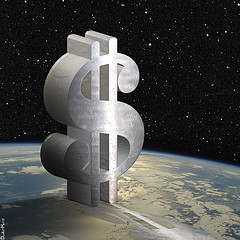flickr.com/donkeyhotey

Leasing an automobile can leave the average consumer feeling flabbergasted by the mathematical equations that unfold before you.  The very basic process can seem like a numeric undertaking that requires an Einstein-like understanding of figures.  That’s just what the leasing companies would like you to believe.

Many of us shy away from the seemingly complicated equations that determine our monthly lease payments, preferring instead to leave the calculations to the dealer.  It’s actually not that difficult.  Once you have a basic understanding of all the figures in calculating your monthly payments, it can all fall into place very nicely.

The key figures in working out monthly lease payments are as follows:

Lease Term:  The number of months involved in your agreement, typically 24 (two years) or 36 (three years).

MSRP:  The manufacturer’s suggested retail price is the actual list or selling value of the car, also called the sticker price.

Money Factor: This is a figure that determines the interest rate on your lease.  You must insist that the dealer disclose this rate before you agree to the lease.

Residual Value: The estimated value of your vehicle when your lease expires.  Again, you should get this figure from the dealer before signing an agreement.

Those are the basic factors involved in calculating your lease payment.  The formula is really quite simple.  Let’s work out the lease payments for a car based on the following terms:

1.  The MSRP (sticker price) of the car is \$20,000, and the money factor is 3.4% (0.0034).
2.  The scheduled lease term is three years, or 36 months.
3.  The estimated residual percentage is 55%.

Step 1:  Calculate the Depreciation Charge

The first step to finding this portion of your monthly lease payment is to calculate the residual value of the car.  To do this, multiply the MSRP by the residual percentage:

\$20,000 x .55 = \$11,000.

Next, deduct the residual value of the car (\$11,000) from the sticker price:

\$20,000 – \$11,000 = \$9,000

This amount is divided by the number of months in the term of the lease:

\$9,000 / 36 = \$250

The resulting amount of \$250 is the first part of your monthly payment amount, called the depreciation charge.

Step 2:  Interest ChargeCheck out the waffle weaves! The world's best car drying towel.The second part of your monthly lease payment is the interest charge, calculated using the money factor.
To calculate this portion of your payment, add the MSRP to the residual value, and multiply by the money factor:

\$20,000 + \$11,000 = \$31,000
\$31,000 x 0.0034 = \$105.4

Step 3:  Add It Up

To find your approximate monthly payment, simply add the depreciation charge to the interest charge:

\$250 + \$105.40 = \$355.40

Finally, we get the approximate monthly payment by adding the two figures together:

\$250 + \$105.4 = \$355.40

When leasing a \$20,000 car over three years with a residual percentage of 55% and a money factor of 3.4%, your estimated lease payment will be about \$355.40 per month.

There are a few steps, but the basic calculations are fairly simple.  Just follow the three steps:

1- Monthly Depreciation Charge:

MSRP x Depreciation Percentage = Residual Value
MSRP – Residual Value = Depreciation over lease term
Depreciation over lease term / lease term (# of months) = monthly depreciation charge

2- Money Factor Charge:

(MSRP + residual value) x money factor  = money factor payment

3- Estimate Monthly Payment:

Depreciation charge + money factor payment = estimated monthly lease payment

It’s important to remember that this simplified calculation does not take additional fees and charges into account.  There may be added charges, like fees and rebates, or discounts including dealer incentives, that will affect the final monthly lease payment amount.  However, the formula can give you a general estimate of what the payments will be on your choice of vehicle.

In order to secure the best possible deal on your lease, you don’t need to have a genius I.Q.; you just have to be familiar with the basic formula.  Don’t assume that your dealer will automatically calculate the most affordable payments.  Lease agents and car dealers are out to make a buck, so you do the math.

Tagged with:

Filed under: Buy A Car